# Ex 13.8 NCERT Solutions- Surface Areas and Volumes Class 9 Notes | EduRev

## Class 9 : Ex 13.8 NCERT Solutions- Surface Areas and Volumes Class 9 Notes | EduRev

The document Ex 13.8 NCERT Solutions- Surface Areas and Volumes Class 9 Notes | EduRev is a part of the Class 9 Course Mathematics (Maths) Class 9.
All you need of Class 9 at this link: Class 9

Note: Assume π = (22/7), unless stated otherwise.

Question 1. Find the volume of a sphere whose radius is (i) 7 cm (ii) 0.63 m
Solution:
(i) Here, radius (r) = 7 cm

∴ Volume of the sphere =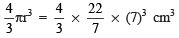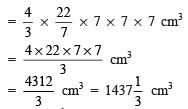Thus, the required volume = 1437 (1/3)cm3

(ii) Here, radius (r) = 0.63 m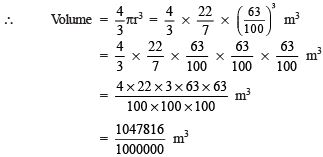= 1.047816 m3 = 1.05 m3 (apπrox)

Thus, the required volume is 1.05 m3 (apπrox).

Question 2. Find the amount of water displaced by a solid spherical ball of diameter (i) 28 cm (ii) 0.21 m
Solution:
(i) Diameter of the ball = 28 cm
∴ Radius of the ball (r) =(28/2) cm = 14 cm

⇒ Volume of the spherical ball =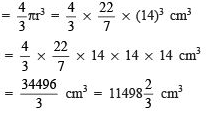Thus, the amount of water displaced = 11498 (2/3) cm3.

(ii) Diameter of the solid spherical ball = 0.21 m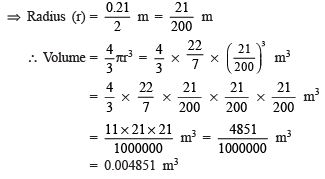Question 3. The diameter of a metallic ball is 4.2 cm. What is the mass of the ball, if the density of the metal is 8.9 g per cm3?
Solution
: Diameter of the metallic ball = 4.2 cm
⇒ Radius (r) = (42/2) cm = 2.1 cm

∴ Volume of the metallic ball =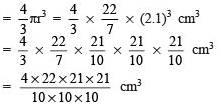∵ Density = 8.9 g per cm3
∴ Mass of the ball = 8.9 x [Volume of the ball]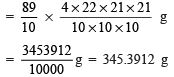= 345.39 g (apπrox.)
Thus, the mass of ball is 345.39 g (apπrox.).

Question 4. The diameter of the moon is apπroximately one-fourth of the diameter of the earth.
What fraction of the volume of the earth is the volume of the moon?
Solution:
Let diameter of the earth be 2r.

⇒ Radius of the earth =(2r/2)  = r
∵ Diameter of the moon = (1/4)(Diameter of the earth)
⇒ Radius of the moon = (1/4) (r) = (r/4)
∴ Volume of the earth = (4/3)πr 3

Volume of the moon =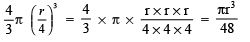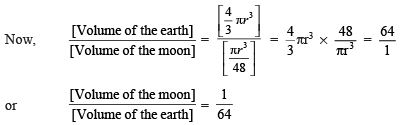∴ The required fraction is (1/64)
.

Question 5. How many litres of milk can a hemispherical bowl of diameter 10.5 cm hold?
Solution:
Diameter of the hemisphere = 10.5 cm
⇒ Radius of the hemisphere (r) = (10.5/2) cm = (105/20) cm
Volume of the hemispherical bowl = (2/3) πr 3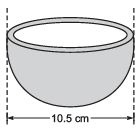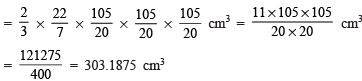∴ Capacity of the hemispherical bowl = 303.1875 cm3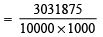= 0.3031875 l = 0.303 l (apπrox.)                              [∵ 1000 cm3 = 1 l]
Thus, the capacity of the bowl = 0.303 l (apπrox.)

Question 6. A hemispherical tank is made up of an iron sheet 1 cm thick. If the inner radius is 1 m, then find the volume of the iron used to make the tank.
Solution:
∵ Thickness = 1 cm = (1/100) m = 0.01 m
∴ Outer radius (R) = 1 m + 0.01 m = 1.01 m
Now, outer volume of the hemispherical bowl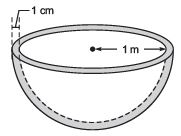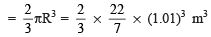Inner volume of the hemispherical bowl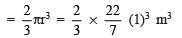∴ Volume of the iron used = [Outer volume] – [Inner volume]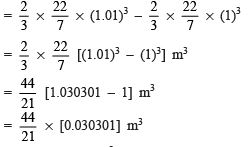= 0.063487776 m3
= 0.06348 m3 (apπrox.)
Thus, the required volume of the iron = 0.06348 m3

Question 7. Find the volume of a sphere whose surface area is 154 cm2
Solution:
Let ‘r’ be the radius of the sphere.
∴ Its surface area = 4πr2
⇒ 4πr= 154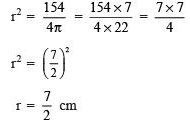Now, volume of the sphere =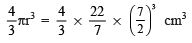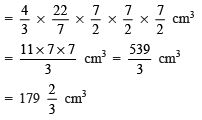Thus, the required volume of the sphere =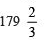cm3

Question 8. A dome of a building is in the form of a hemisphere. From inside, it was white washed at the cost of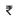4989.60. If the cost of white washing is20 per square metre, find the (i) inside surface area of the dome, (ii) volume of the air inside the dome.
Solution:
Total cost of white washing =4989.60
Rate of white washing =20 per m

∴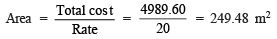Let ‘r’ be the radius of the hemispherical dome.
∴ Surface area = 2πr2
∴   2πr2 = 249.48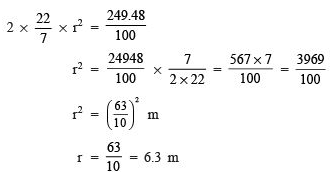(i) To find the inside surface area of the dome:
∵ Radius of the hemisphere (r) = 6.3 m
Surface area of a hemisphere = 2πr2 m2
∴ Surface area of the dome = 2 x (22/7) (6.3)2 m2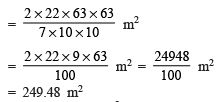Thus, the required surface area of the dome = 249.48 m

(ii) To find the volume of air in the dome:
Volume of a hemisphere = (2/3)πr 3

∴ Volume of the dome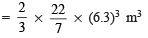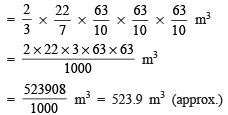Thus, the required volume of air inside the dome is 523.9 m3 (apπrox.).

Question 9. Twenty seven solid iron spheres, each of radius r and surface area S are melted to form a sphere with surface area S'. Find the (i) radius r’ of the sphere, (ii) ratio of S and S'.
Solution:
(i) To find r ':
∵ Radius of a small sphere = r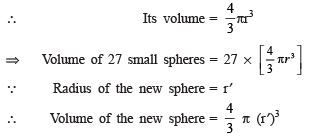Since,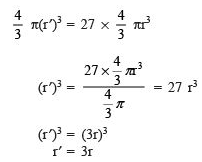(ii) To find ratio of S and S ':
∵ Surface area of a small sphere = 4πr
∴ S= 4πrand S ' = 4p(3r)2

Now,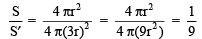Thus, S : S' = 1 : 9

Question 10. A capsule of medicine is in the shape of a sphere of diameter 3.5 mm. How much medicine (in mm3) is needed to fill this capsule?
Solution:
Diameter of the spherical capsule = 3.5 mm
⇒ Radius of the spherical capsule (r) = (3.5/2) mm
∴ Volume of the spherical capsule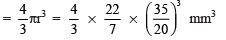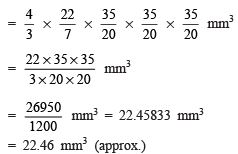Thus, the required quantity of medicine = 22.46 mm3 (apπrox).

Offer running on EduRev: Apply code STAYHOME200 to get INR 200 off on our premium plan EduRev Infinity!

## Mathematics (Maths) Class 9

190 videos|233 docs|82 tests

,

,

,

,

,

,

,

,

,

,

,

,

,

,

,

,

,

,

,

,

,

;